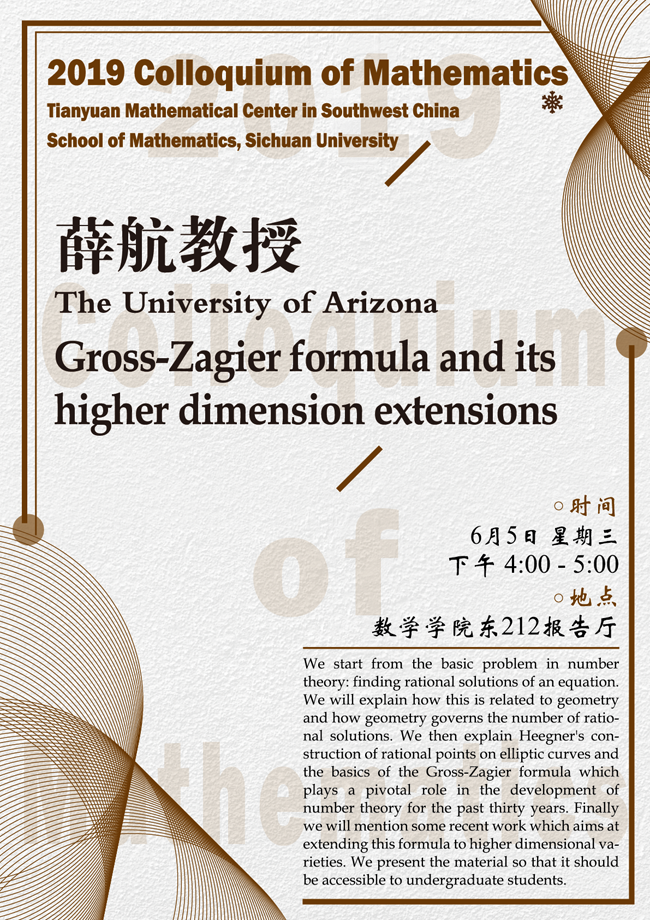### Gross-Zagier formula and its higher dimension extensions

#### E212  School of Mathematics#### ABSTRACT

We start from the basic problem in number theory: finding rational solutions of an equation. We will explain how this is related to geometry and how geometry governs the number of rational solutions. We then explain Heegner's construction of rational points on elliptic curves and the basics of the Gross-Zagier formula which plays a pivotal role in the development of number theory for the past thirty years. Finally we will mention some recent work which aims at extending this formula to higher dimensional varieties. We present the material so that it should be accessible to undergraduate students.

#### SUPPORTED BY

Tianyuan Mathematical Center in Southwest China

School of Mathematics, Sichuan University